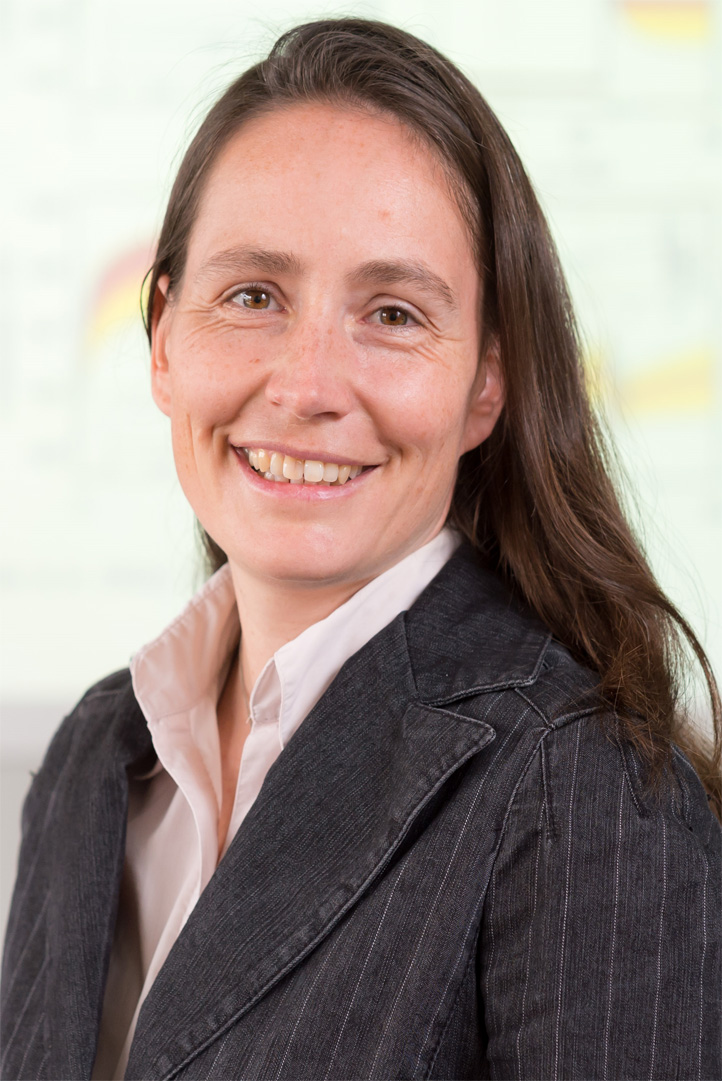# Mathematical modeling and simulation of cellular systems

All models are wrong, but some are useful! (George Box)

## Systems Biology

Systems Biology is an interdisciplinary approach with the goal to increase the understanding of biological systems. It is a consequential application of tools and methods from systems and control engineering to biological systems. At the heart of this approach is the development of mathematical models to simulate and analyze cellular systems.

The biological systems under investigation range from intracellular processes like signal transduction in mammalian cells to cell-cell interactions in specific tissues like a tumor. While biological expertise is introduced by a number of long-term collaborations with experimental biologists, the mathematical modeling of the considered processes is done in our group. The wide range of applications is reflected by the diverse modeling frameworks that are applied, including stochastic models, ordinary differential equations, models where only the network structure is considered, and models for heterogeneous cell populations.

Mathematical analysis methods aim at a mechanistic understanding of the underlying processes and, based on this, at deriving model based predictions and hypotheses for new scenarios. The ISA systems biology group focuses on applied projects and on the development of model analysis methods that are inspired by statistical learning methods and control engineering. In order to support the modeling process, parameter identification from experimental data is a very relevant issue. In our group, various new approaches to solve this problem are being studied. Models for biological systems are often subject to a large degree of uncertainty. To deal with this problem, we develop methods for uncertainty and robustness quantification which allow deriving reliable statements from uncertain models. A specific interest of the ISA systems biology group also lies in the analysis of complex dynamical behavior of biological systems, such as the switch-like behavior encountered in bi-stable systems.

Group members: Nicole Radde, Viviane KlingelVincent Wagner, Amatus Beyer and Sebastian Höpfl.

### Contact